No Meaning Left Unlearned: Predicting Learners’ Knowledge of Atypical Meanings of Words from Vocabulary Tests for Their Typical Meanings
Tokyo Gakugei University
ehara@u-gakugei.ac.jp

# ABSTRACT

Language learners are underserved if there are unlearned meanings of a word that they think they have already learned. For example, “circle” as a noun is well known, whereas its use as a verb is not. For artificial-intelligence-based support systems for learning vocabulary, assessing each learner’s knowledge of such atypical but common meanings of words is desirable. However, most vocabulary tests only test the typical meanings of words, and the texts used in the test questions are too short to apply readability formulae. We tackle this problem by proposing a novel dataset and a flexible model. First, we constructed a reliable vocabulary test in which learners answered questions regarding typical and atypical meanings of words. Second, we proposed a simple but powerful method for applying flexible and context-aware masked language models (MLMs) to learners’ answers in the abovementioned vocabulary test results. This is a personalized prediction task, in which the results vary among learners for the same test question. By introducing special tokens that represent each learner, our method can reduce the personalized prediction task to a simple sequence classification task in which MLMs are applicable. In the evaluation, item response theory (IRT)-based methods, which cannot leverage the semantics of test questions, were used as baselines. The experimental results show that our method consistently and significantly outperformed the IRT-based baselines. Moreover, our method is highly interpretable because one can obtain the learners’ language abilities from the first principal component scores of the token embeddings representing each learner.

# Keywords

Second Language Learning, Item Response Theory, Masked Language Models, Natural Language Processing

# 1. INTRODUCTION

In intelligent tutoring systems, it is important to accurately identify what is known and what is unknown to the learner to recommend learning items according to the learner’s characteristics, such as the learner’s ability. When there are many learning items, it is difficult to test them all. In this case, only some of the knowledge is tested, and the test results are used to predict the other held knowledge.

This is especially fitting to vocabulary learning support systems for foreign languages. Because of the large vocabulary of a foreign language, research has been conducted in the fields of natural language processing (NLP) and applied linguistics to test only some words and to predict the held knowledge of other words based on the test results . As a result, standardized test questions have been developed to test learners’ knowledge.

However, for polysemous words, where a word has multiple meanings, the task of testing knowledge of only typical meanings and predicting the held knowledge of unexpected meanings from only the test results is a challenge. Because polysemous words are especially common in high-frequency words (words with high frequency in large corpora) that foreign language learners learn early on, it is also important for learners to properly grasp and understand the meaning of these words in context. However, many studies on foreign language learning support have focused on vocabulary size, and this area has been relatively unexplored. To the best of our knowledge, standardized questions that test the meanings of polysemous words have not been developed.

In the case of polysemous words, it is difficult to test for meanings other than the most typical ones. This can be attributed to the large number of words that must be learned; testing a learner’s knowledge of a single word more than once would impose a greater testing burden on learners. Hence, learners may often fail to learn word meanings other than the most representative meaning.

To improve learners’ language abilities, it is important to ensure that they learn all major meanings of polysemous words. In the development of artificial intelligence (AI)-based systems that support language learners by identifying and recommending such unlearned meanings, it is essential to identify the meanings of polysemous words that a given learner already knows. However, considering the testing burden, sufficient time is available to test only a few additional word meanings. Given that vocabulary tests use numerous words to measure a learner’s overall vocabulary, most typical vocabulary tests query only the major meanings of polysemous words. Therefore, it is desirable to be able to predict the extent to which a learner knows the non-representative meanings of a word by using existing test results for major word meanings. For example, it should be possible to predict whether a learner who knows the meaning of “figure” referring to a number also knows the meaning of “figure” referring to a person.

Intuitively, one can easily think of a two-step approach in which one first predicts the meaning of a polysemous word in a running sentence and then assigns the difficulty of each meaning of a word. However, this approach is impractical because the categorization of meanings by linguists is not typically designed for language learning. For example, Figure 1 show examples of the word “period” used in distinctly different contexts. However, linguistic categorization can be too fine-grained for language learners. For example, WordNet , one of the most carefully designed thesauri for the English language, separately lists “period” as a geological period as being a different meaning from “period” as a timespan. This is counter-intuitive for language learners and impractical for estimating the difficulty of “period” as a geological period separately from that as a timespan. Hence, another approach is necessary in which one directly predicts how likely it is that the language learner understands the meaning of a word used in an input sentence.

To the best of our knowledge, no existing datasets or methods have been provided in the literature to evaluate the extent to which such problems can be solved. Because it is presumably difficult to capture the meanings of words in running texts, these types of questions have not been extensively studied in vocabulary testing studies in applied linguistics . Therefore, in this study, we propose a dataset and methods to evaluate how well these problems can be solved. Figure 1 are examples of the dataset. To this end, we used deep transfer learning state-of-the-art neural language models (MLMs), namely masked language models such as bidirectional encoder representations from transformers (BERT) . Deep-learning-based NLP techniques cannot simply be applied to this personalized prediction problem in which different predictions must be made for each learner, even for the same given input sentence. Although recent educational AI studies also used BERT , they did not address this issue because they do not deal with personalized prediction tests.

In the proposed method, we demonstrate a simple approach to reduce this personalized prediction problem to a typical sequence classification problem in NLP by adding special tokens that represent learners in language models. In our experiments, the prediction performance of the proposed method was superior to that of other methods such as item response theory (IRT) by a statistically significant margin.

Our method also showed high interpretability matching that of the IRT models: one merit of using IRT models is that they are highly interpretable, e.g., capable of extracting the ability estimates from the model, which BERT models cannot do. We showed that the first principal component scores of the embedding of the tokens representing each learner had a statistically significant correlation with the learners’ ability values obtained using the IRT.

The contributions of this paper are as follows.

• We focused on the importance of predicting whether language learners know the atypical meanings of a word and developed an evaluation dataset for this purpose.
• In addition, we proposed a simple method for applying deep transfer learning techniques to the aforementioned personalized prediction problem by introducing tokens that represent learners. The prediction performance of the proposed method is superior to that of IRT by a statistically significant margin.
• Finally, we demonstrated that with the proposed method, we can easily obtain learners’ ability values by using the first principal component scores of token embedding. This indicates that our method is highly interpretable, and hence suitable for educational use.

# 2. RELATED WORK

In educational data mining,  models the spaced repetition, or students’ memorization of learning items repeatedly, by extending statistical models. While  focuses on the temporal aspect in the process of learning second language vocabulary, we focus on predicting each learner’s knowledge of atypical meanings of words from the test results of typical meanings of words, considering text semantics and contexts. In AI in education,  addressed a case study of the personalized English vocabulary learning of 37 Syrian refugees using a language learning application called SCROLL. This study did not address the methodology or algorithm used in SCROLL but the case study of using it. While  applied BERT to readability prediction,  was not personalized.

Vocabulary test datasets were previously published via self-report testing , or multiple-choice testing . However, to the best of our knowledge, no reliable vocabulary test datasets in which typical and atypical meanings of words have both been tested have been published.

Other datasets seemingly similar to our dataset include the SLAM dataset , which is based on responses to questions on the language learning application Duolingo, and the Complex Word Identification (CWI) dataset , where a large number of language learners were asked to annotate unfamiliar words in a sentence. The difference between these datasets and ours is that each subject answered only a small portion of the many questions.

# 3. VOCABULARY TESTING DATASETS

This section elaborates on our dataset.  is a publicly available dataset of vocabulary test results of language learners. However, it does not include questions regarding any typical/atypical meanings of words. Nevertheless, for comparability, we adopted their settings to build our dataset. Our dataset was compiled from the crowdsourcing service Lancers 1. To find learners who had some interest in learning English, only learners who had taken the Test of English for International Communication (TOEIC) test 2 in the past were permitted to take the vocabulary test. As a result, 235 subjects responded to the questionnaire. Because most learners in Lancers are native Japanese speakers, the native language of the learners was also assumed to be Japanese.

For typical vocabulary test questions, we used vocabulary size test (VST) , as  did. However, unlike , our focus is highly frequent words. In VST, the questions are ordered by the frequency of the corpus that  used for making VST. To reduce the test burden on the participants and to easily collect accurate answers, we eliminated 30 low-frequency words from the test. The remaining 70 questions were used for a typical vocabulary test. An example of these questions is shown in Figure 1 (a). The word(s) being tested are underlined in the sentence. The subject is asked to choose the option that is closest in meaning to the original sentence when the word(s) being tested are replaced. All options were designed to be grammatical when replaced.

We developed 13 questions that tested atypical meanings of words as follows. First, a computer science researcher, who was a non-native but fluent English speaker, drafted the questions. Second, English professors, namely two native English speakers (i.e., an American English speaker and an Australian English speaker) and a non-native speaker, checked and edited the questions for validity. In the actual examples of these two test sets in Figure 1 (b), the word “period” has a physiological meaning in addition to the usual meaning as a timespan. The subjects were asked to answer $13$ questions, such as Figure 1 (b) before the $70$ lexical test of typical usage. We included one question with an unexpectedmeaning but without a corresponding question on the group of typical word meaning questions. Therefore, the number of question pairs was $12$. More details of our dataset are provided in the Appendix.

# 4. ITEM RESPONSE THEORY

This section briefly describes the IRT models. Let the number of subjects be $J$ and the number of questions (or items) be $I$. For simplicity, we identify the index of the subject with the subject and the index of the item with the item. For example, the $I$-th item is simply written as $I$. We assume that ${y}_{\mathit{ij}}$ is $1$ when subject $j$ answers item $i$ correctly, and $0$ when the subject answers incorrectly. Given the test result data $\left\{{y}_{\mathit{ij}}|i\in \left\{1,\dots ,I\right\},j\in \left\{1,\dots ,J\right\}\right\}$, the 2PL model models the probability that subject $j$ answers item $i$ correctly with the following equation

$P\left({y}_{\mathit{ij}}=1|i,j\right)=\sigma \left({a}_{i}\left({𝜃}_{j}-{d}_{i}\right)\right)$(1)

Here, $\sigma$ is the logistic sigmoid function defined as $\sigma \left(x\right)=\frac{1}{1+\mathrm{exp}\left(-x\right)}$. where the $\sigma$ is a monotonically increasing function with $\left(0,1\right)$ as its value range and $\sigma \left(0\right)=0.5$. The $\sigma \left(x\right)$ is used to project real numbers into the range of $\left(0,1\right)$ and treat them as probabilities. In (1), ${𝜃}_{j}$ is called the ability parameter, and is a parameter that represents the ability of the subject. ${d}_{i}$ is the difficulty parameter representing the difficulty of the item. From (1), when ${𝜃}_{j}$ is greater than ${d}_{i}$, the probability of the subject answering correctly is higher than that of answering incorrectly. The value ${a}_{i}>0$ is usually positive and is called the discrimination parameter. The larger this value, the more ${𝜃}_{j}-{d}_{i}$ affects the probability of correct or incorrect answers. It is called “discrimination” because ${𝜃}_{j}-{d}_{i}$ makes it easier to distinguish whether subject $j$ will answer question $i$ correctly or not. More intuitively, this indicates that question $i$ is a good question in that it can accurately distinguish between learners with high ability and those with low ability.

# 5. PROPOSED METHOD

## 5.1 Deep Transfer Learning

In this section, we describe our proposed method. The proposed method is based on Transformer models such as BERT . Transformer models employ transfer learning to capture the semantics of texts written in natural language Figure 2. First, a pre-trained model is prepared using large raw (i.e., unannotated) texts. This model can be trained using raw texts written by native English speakers, such as the Wikipedia text. This procedure is called pre-training. Typically, pre-training incurs high computational costs. Hence, we downloaded and prepared publicly available pre-trained models. Each model is identified by an ID such as bert-large-cased, which denotes that the model was trained on a large Wikipedia corpus in a case-sensitive manner. Importantly, a pre-trained model can be used for many tests depending on the fine-tuning.

Then, an additional fine-tuning is performed to train the pre-trained model to the intended task. To this end, a small annotated corpus must be prepared for use in a supervised learning procedure. Such corpora are generally costly to construct; in this case, the corpus comprised the results of a vocabulary test. After the model is fine-tuned, it can be used to make predictions based on new input sentences.

## 5.2 Reducing the Personalized Prediction into Sequence Classification

Given a language learner taking a test and a word in a sentence, as shown in Figure 1 (b), our goal is to predict whether the test-taker knows the word. Notably, this is a personalized prediction; the prediction results differ among individual learners. In contrast, deep transfer learning does not support personalized predictions. Publicly available pre-trained models are preferable because pre-training is costly. Moreover, designing a new model that can achieve high performance using the available pre-trained models is relatively difficult. Thus, reducing the personalized prediction problem to a typical NLP task can be a practical solution, instead of developing a novel neural model for this task.

We reduced the personalized prediction task into a sequence classification task as shown in Figure 3. Sequence classification is a task in which a classifier takes a sequence or text as the input and predicts its label. Hence, to train the classifier, pairs of text and associated labels are used to constitute a small corpus for supervised learning in the fine-tuning phase. Thus, to use sequence classifiers in this task, we first need to convert the original vocabulary test result dataset into a sequence classification dataset so that sequence classifiers can handle the dataset.

In the example of Figure 3, USR3, the test-taker whose ID was 3 answered correctly on a multiple-choice question of the typical meaning of the word “period,” but incorrectly answered a question on the atypical meaning. To convert this record into a format that accepts a sequence classifier, we added special tokens: [USRn].

Here, [USRn], where $n$ is replaced by the test-taker ID, represents each test-taker, or learner (user). By placing this at the beginning of the sequence, we notify the classifier that we want to predict the response of the test-taker specified by this token. Therefore, the example in Figure 3 shows that we aim to predict the response of USR3 to the sequence “It is a difficult period.”. In this example, as USR3 answered the multiple-choice question correctly, the label for the question was set to 1. The rationale behind this conversion is that the test-taker could read the sentence if the test-taker answered the question correctly. Hence, the label denotes that the test-taker was able to successfully read the short sentence “It is a difficult period.”

Likewise, USR3’s answer to an atypical question can be converted to the sequence on the right-hand side of Figure 3. In this example, as USR3 answered incorrectly, the label for the question was set to $0$. The option that is incorrectly chosen by USR3 (“hour” in the example of Figure 3) is ignored in the sequence. This ensures a fair and accurate comparison, because IRT-based methods also do not consider the incorrect options or distractors chosen by the test-taker. Rather, they only consider whether a test-taker chooses the correct option. As each of these tokens represents a test-taker, there are as many tokens as the number of test-takers, starting from [USR1].

Thus, the dataset can be converted into a sequence classification format. In the transformers library, the tokens used for Transformer models can be added using the add_tokens function. After conversion, we simply use the AutoModelForSequenceClassification to construct sequence classifiers. Note that this conversion enables BERT to handle personalized prediction by introducing [USRn] tokens. We introduce special tokens for each user and insert the token into the beginning of the sentence.

# 6. IRT-BASED ANALYSIS

To obtain the difficulty and discrimination parameters for IRT, we used the pyirt Python library 3. This library was developed to conduct IRT analyses using marginalized maximum likelihood estimation (MMLE) . For the dataset described above, we used the 2PL model to obtain the abovementioned parameters. The dataset includes 12 pairs of questions, such as Figure 1. The difficulty parameters for the usual and unexpected examples are shown on the horizontal and vertical axes, respectively, and plotted at the same scale and range on the horizontal and vertical axes in Figure 4. Each point represents a single word.

A dotted diagonal line is shown from the lower left to the upper right of Figure 4. The horizontal and vertical axes of Figure 4 represent the values of the difficulty parameter; the higher the value, the more difficult the task was judged to be. The point to the upper left of the diagonal line indicates that the difficulty level of an example that seemed unexpected to the learner was higher than that of the typical example. Moreover, the word was judged to be more difficult for the learner to correctly answer questions from the vocabulary test data. The results of the Wilcoxon test showed that the column of values on the vertical axis was larger than that on the horizontal axis by a statistically significant margin ($p<0.01$), suggesting that the vertical-axis questions were more difficult than the horizontal-axis questions.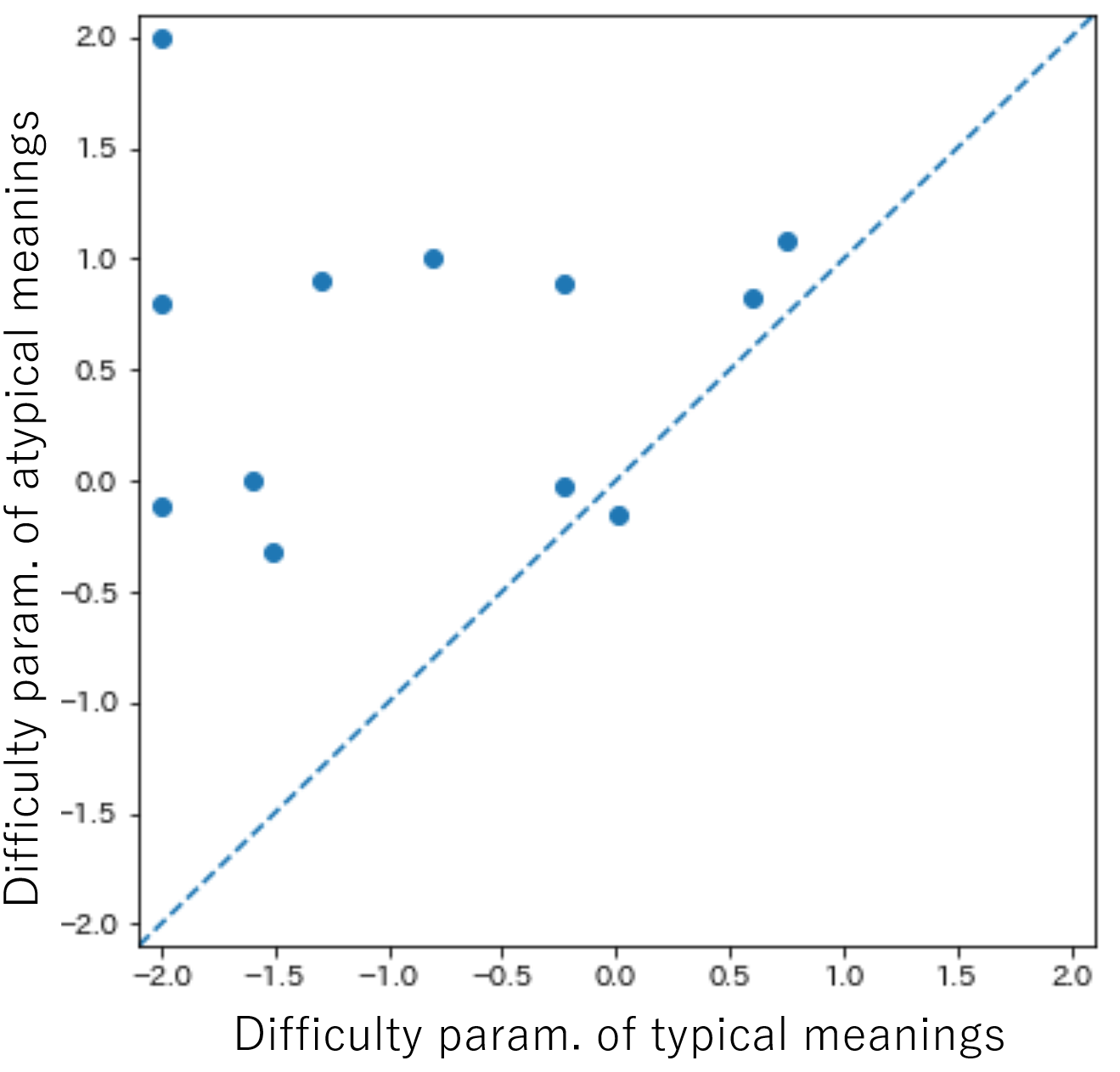Figure 4: Plot of the difficulty of a typical meaning (horizontal) vs. that of an atypical meaning (vertical) for each word.

Discrimination was also analyzed. The plot is omitted for space limitation. Atypical meanings are expected to be less discriminating than typical meanings, because even high-ability learners may not know the correct answers of atypical meanings, whereas low-ability learners may know them. This tendency was observed as follows: For all words, it was estimated that the discrimination of typical examples was higher than that of unexpected examples. This result was found to be statistically significant using the Wilcoxon test ($p<0.01$).

# 7. EXPERIMENTS OF PREDICTIONS

## 7.1 Item Response Theory-Based Settings

A naive method to deal with the difficulty of an atypical meaning of a word is to simply regard its difficulty as being the same as that of its typical meaning. Hence, we measured the negative effects of substituting the difficulty of the atypical meanings of a word with the difficulty of its typical meanings on predicting the subjects’ responses.

To investigate this, we conducted the following experiment, as shown in Figure 5. First, we divided 235 subjects into 135 and 100 subjects. We estimated the parameters without using the responses of the latter 100 subjects for the 12 questions (1,200 responses). The parameters of the 12 atypical example questions were estimated only from the responses of the former 135 subjects, while the parameters of the 70 typical example questions were estimated from the responses of all 235 subjects. From (1), we can see that the estimated values of subject ability ${𝜃}_{j}$ and the difficulty of the example ${d}_{i}$ are sufficient to predict if subject $j$ answers question $i$ correctly or not by checking if ${𝜃}_{j}>{d}_{i}$ or not, respectively. Hence, once all $\mathit{thet}{a}_{j}$ and ${d}_{i}$ can be estimated, we can make predictions. For the 12 typical and atypical question pairs, we have two prediction methods: one that uses the difficulty parameters of the typical examples of the pairs for ${d}_{i}$ and one that uses that of atypical examples of the pairs. Thus, we compared the prediction accuracy of these 1,200 responses.

Several methods are conventionally used to estimate the parameters of IRT models. As in the previous sections, we used the pyirt library, which implements MMLE, for parameter estimation. MMLE assumes that the ability parameter can only take several values to marginalize. This causes stepwise shapes in the resulting ability parameter plots.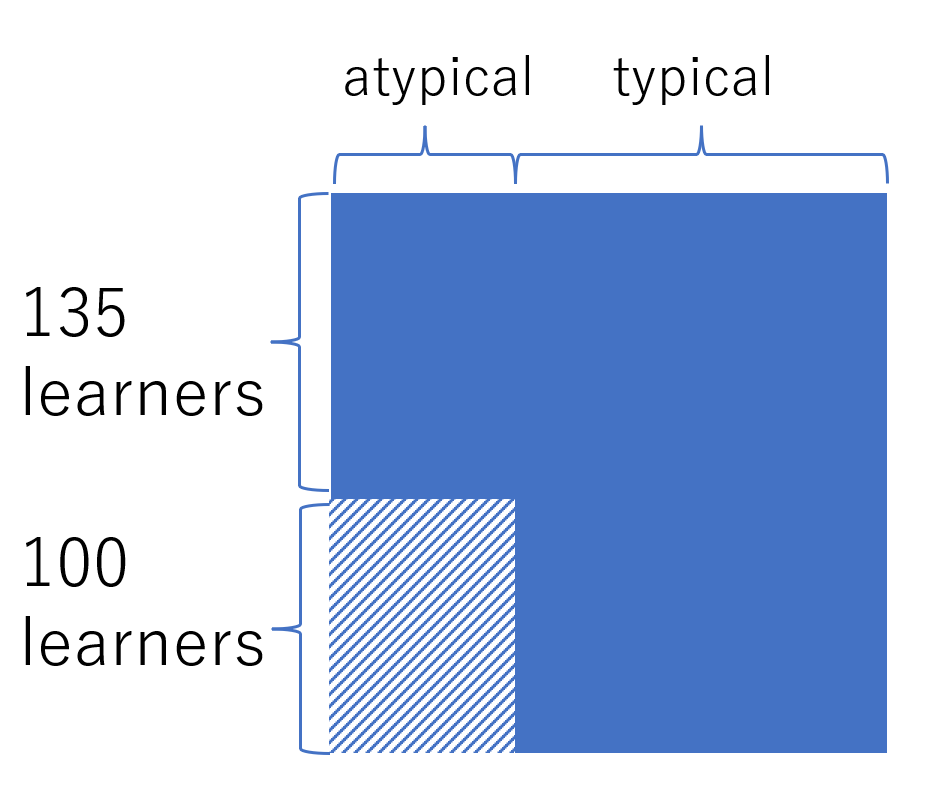Figure 5: Experiment setting. Filled areas are training data, i.e., used for estimating the parameters. All methods are evaluated based on the accuracy of the responses of the dashed area, i.e, the responses for atypical words of the 100 test learners.

## 7.2 Our Settings

We constructed a BERT-based personalized predictor . For the neural classification, we used the same settings described in Section 5.2. As described in Table 1, we compared the pre-trained Transformer models, all of which were publicly available from the HuggingFace website 4. Then, we conducted a fine-tuning by using our own data.

Table 1: Transformer Models Used for Experiments
Model Name Model cased/uncased
bert-base-cased BERT  cased
bert-base-uncasedBERT  uncased
bert-large-cased BERT  cased
roberta-base RoBERTa cased
albert-base-v2 ALBERT  uncased

Here, we describe how we converted the personalized prediction of whether a learner knew a given word into the task of sequence classification and its fine-tuning. Sequence classification is a supervised classification task in which the goal is to predict labels by using a sequence as an input. Here, the label is $1$ if the learner knows the meaning of the word, i.e., answered the question about the meaning of the word correctly; otherwise, the label is $0$.

Because the aim is to make personalized predictions, it is necessary to incorporate learner test-takers in the sequence. Thus, we added special tokens to represents individual learners. For example, if the sequence starts from “[USR3]”, this means that we want to predict whether the learner with ID 3 can read the sentences that follow. Hence, “[USR3] It was a difficult period.” asks if the learner with ID 3 could read the sentence “It was a difficult period.”. The goal of the task is to predict $1$ or $0$, where $1$ indicates that the learner could read the specified sentence, and $0$ indicates that the learner was unable to do so. We fine-tuned the pre-trained BERT model in this manner using the “training” data shown in Figure 5. For the estimation, we used the Adam optimizer , in which the batch size was 32.

Table 2: Predictive Accuracies of the Dashed Area
Base Method Accuracy
IRT (ability - diffcl. of typical word) 0.544
IRT (ability - diffcl. of atypical word)0.644
OURS (bert-large-cased) 0.674 (**)
OURS (bert-base-cased) 0.688 (**)
OURS (bert-based-uncased) 0.655
OURS (roberta-base) 0.681 (**)
OURS (albert-base-v2) 0.671 (*)

## 7.3 Results

Table 2 shows the predictive accuracies of all methods. The results showed that the prediction accuracy of the direct method was $64.4%$ and that of the alternative method was $54.4%$, a difference of $10$ points. This difference was significant at $p<0.01$ in the Wilcoxon test. This result indicates that estimating the difficulty of atypical meanings of words from those of typical words is a challenging task.

Our model achieved the best performance among the listed models. The name in () indicates the pre-trained model used for the experiments. In particular, our model significantly outperformed the IRT models with the best accuracy. This result was also statistically significant using the Wilcoxon test ($p<0.01$). This is denoted by (**) in Table 2. As BERT considers the semantics of the question, this result suggests that the traits of each learner test-taker was captured via the embeddings of the $\left[\mathit{USR}\right]$ tokens during the fine-tuning.

Table 2 showed the best performance for bert-base-cased. bert-base-cased achieved a performance better than bert-large-cased. The reasons of this are presumably as follows. Although the word embeddings of Transformer models are trained by many examples in their pre-trained corpus, the word embedding vectors of learner tokens, which represent learners’ characteristics, such as their abilities, were trained solely on a relatively small training data in the fine-tuning phase. Obviously, no learner tokens appeared in the pre-trained corpus. Hence, it could be possible that bert-large-cased has too many parameters to be tuned using the small training data in fine-tuning compared with bert-base-cased.

Table 2 also shows that a model must be cased to achieve a good accuracy, considering that roberta-base is cased whereas albert-base-v2 is uncased. This is presumably because the model needs to recognize the start of a sentence, which starts with a capitalized word, since each question consists of a short sentence in this experimental setting.

# 8. EXTRACTING ABILITY VALUES

As stated above, our method handles learners as tokens. Transformer-based methods internally use “word embeddings” that represents the meaning of the tokens in the form of vectors. Hence, by obtaining the word embeddings of the learner tokens that we introduced, it was possible to analyze learners’ characteristics, such as their language abilities.

As word embeddings are typically multi-dimensional, dimension reduction methods such as principal component analysis (PCA), can be used to obtain abilities from the embeddings of learner tokens. Figure 6 shows the plot of a PCA of the ability parameters of test-takers of the vocabulary test dataset against the first principal component scores of each token of the token embeddings in the case of bert-large-cased in Table 2. A clear correlation can be observed between the two. The correlation coefficient was $0.72$, and was statistically significant ($p<0.01$). In this manner, learners’ abilities can be obtained through PCA of the test-taker tokens in our method, which means that our method is equipped with the interpretability of IRT models.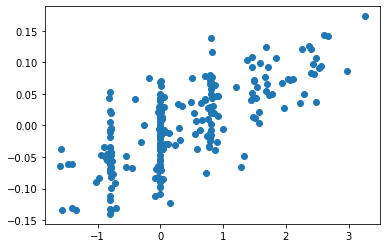Figure 6: Relationship between IRT ability parameters estimated by pyirt (horizontal) and the first principal component of the learner token embeddings (vertical)

In Figure 6, we used pyirt for estimating the ability parameters. To check the correlation using IRT software other than pyirt, following a standard textbook for educational psychology , we also conducted experiments using the R “ltm” package, which was developed completely independently of pyirt. Unlike pyirt, which uses MMLE, ltm uses the expected a posteriori (EAP) method for parameter estimation. Again, a statistically significant correlation was observed: the Pearson’s correlation was also $0.72$, ($p<0.01$).

# 9. CONCLUSION

In this study, we tackled the task of predicting whether language learners know the atypical meanings of a word and developed an evaluation dataset for this purpose. We proposed a simple method for applying MLMs to the aforementioned personalized prediction problem by introducing tokens that represent learners. The prediction performance of the proposed method was superior to that of IRT by a statistically significant margin. We also showed that, with the proposed method, one can easily obtain learners’ ability values using the first principal component scores of token embeddings. This result indicates that our method is highly interpretable and, hence, suitable for educational use.

The learner token embeddings that we introduced are multi-dimensional. While we showed that the first principal component score significantly correlated with the test-taker’s ability parameter, the other components may encode the learner’s other types of ability. In IRT, there is a similar idea to model the learner’s ability as a multidimensional vector, called “multidimensional IRT”. Our future work is to compare the other principal components of learner token embeddings with multidimensional IRT.

# 10. ACKNOWLEDGMENTS

This work was supported by ACT-X Grant Number JPMJAX2006 and JSPS KAKENHI Grant Number 18K18118, Japan. We used the ABCI infrastructure of AIST and the miniRaiden system of RIKEN for the computational resources. We thank Assoc. Prof. Joy Taniguchi and other members of the Shizuoka Institute of Science and Technology for their cooperation in preparing the dataset. We appreciate the anonymous reviewers’ valuable comments.

# 11. REFERENCES

1. V. Abou-Khalil, B. Flanagan, and H. Ogata. Personal Vocabulary Recommendation to Support Real Life Needs. In I. Roll, D. McNamara, S. Sosnovsky, R. Luckin, and V. Dimitrova, editors, Artificial Intelligence in Education, Lecture Notes in Computer Science, pages 18–23, Cham, 2021. Springer International Publishing.
2. F. B. Baker. Item Response Theory : Parameter Estimation Techniques, Second Edition. CRC Press, July 2004.
3. D. Beglar and P. Nation. A vocabulary size test. The Language Teacher, 31(7):9–13, 2007.
4. B. Choffin, F. Popineau, Y. Bourda, and J.-J. Vie. Das3h: modeling student learning and forgetting for optimally scheduling distributed practice of skills. In Proc. of EDM, 2019.
5. J. Devlin, M.-W. Chang, K. Lee, and K. Toutanova. BERT: Pre-training of Deep Bidirectional Transformers for Language Understanding. In Proc. of NAACL, 2019.
6. Y. Ehara. Building an English Vocabulary Knowledge Dataset of Japanese English-as-a-Second-Language Learners Using Crowdsourcing. In Proc. of LREC, May 2018.
7. Y. Ehara. Lurat: a lightweight unsupervised automatic readability assessment toolkit for second language learners. In Proc. of ICTAI, pages 806–814, 2021.
8. Y. Ehara, Y. Baba, M. Utiyama, and E. Sumita. Assessing Translation Ability through Vocabulary Ability Assessment. In Proc. of IJCAI, 2016.
9. Y. Ehara, Y. Miyao, H. Oiwa, I. Sato, and H. Nakagawa. Formalizing Word Sampling for Vocabulary Prediction as Graph-based Active Learning. In Proc. of EMNLP, pages 1374–1384, 2014.
10. Y. Ehara, I. Sato, H. Oiwa, and H. Nakagawa. Mining Words in the Minds of Second Language Learners: Learner-Specific Word Difficulty. In Proceedings of COLING 2012, pages 799–814, Mumbai, India, Dec. 2012. The COLING 2012 Organizing Committee.
11. Y. Ehara, N. Shimizu, T. Ninomiya, and H. Nakagawa. Personalized Reading Support for Second-language Web Documents. ACM Trans. Intell. Syst. Technol., 4(2):31:1–31:19, Apr. 2013.
12. C. Fellbaum. Wordnet. In Theory and applications of ontology: computer applications, pages 231–243. Springer, 2010.
13. D. P. Kingma and J. Ba. Adam: A method for stochastic optimization. In Proc. of ICLR, 2015.
14. Z. Lan, M. Chen, S. Goodman, K. Gimpel, P. Sharma, and R. Soricut. ALBERT: A lite BERT for self-supervised learning of language representations. CoRR, abs/1909.11942, 2019.
15. B. Laufer and G. C. Ravenhorst-Kalovski. Lexical Threshold Revisited: Lexical Text Coverage, Learners’ Vocabulary Size and Reading Comprehension. Reading in a Foreign Language, 22(1):15–30, Apr. 2010.
16. J. Lee and C. Y. Yeung. Automatic prediction of vocabulary knowledge for learners of Chinese as a foreign language. In 2018 2nd International Conference on Natural Language and Speech Processing (ICNLSP), pages 1–4, Apr. 2018.
17. Y. Liu, M. Ott, N. Goyal, J. Du, M. Joshi, D. Chen, O. Levy, M. Lewis, L. Zettlemoyer, and V. Stoyanov. Roberta: A robustly optimized BERT pretraining approach. CoRR, abs/1907.11692, 2019.
18. M. Maddela and W. Xu. A word-complexity lexicon and a neural readability ranking model for lexical simplification. In Proc. of EMNLP, pages 3749–3760, Oct.-Nov. 2018.
19. I. Nation. How Large a Vocabulary is Needed For Reading and Listening? Canadian Modern Language Review, 63(1):59–82, Oct. 2006.
20. I. S. P. Nation and R. Waring. Teaching Extensive Reading in Another Language. Routledge, Nov. 2019. Google-Books-ID: xRu_DwAAQBAJ.
21. I. Paek and K. Cole. Using R for item response theory model applications. Routledge, 2019.
22. B. Settles. Data for the 2018 Duolingo Shared Task on Second Language Acquisition Modeling (SLAM), 2018.
23. L. Sha, M. Rakovic, A. Whitelock-Wainwright, D. Carroll, V. M. Yew, D. Gasevic, and G. Chen. Assessing algorithmic fairness in automatic classifiers of educational forum posts. In Proc. of AIED, pages 381–394. Springer, 2021.
24. J. T. Shen, M. Yamashita, E. Prihar, N. Heffernan, X. Wu, S. McGrew, and D. Lee. Classifying math knowledge components via task-adaptive pre-trained bert. In Proc. of AIED, pages 408–419. Springer, 2021.
25. S. Xu, G. Xu, P. Jia, W. Ding, Z. Wu, and Z. Liu. Automatic task requirements writing evaluation via machine reading comprehension. In Proc. of AIED, pages 446–458. Springer, 2021.
26. S. M. Yimam, C. Biemann, S. Malmasi, G. Paetzold, L. Specia, S. Štajner, A. Tack, and M. Zampieri. A Report on the Complex Word Identification Shared Task 2018. In Proceedings of the Thirteenth Workshop on Innovative Use of NLP for Building Educational Applications, pages 66–78, New Orleans, Louisiana, June 2018. Association for Computational Linguistics.

# A. DATASETS

The dataset used in this paper will be publicly available. Details of the dataset will be available at http://yoehara.com/ or http://readability.jp/. Some previous datasets such as  are available at http://yoehara.com/.

# B. DISCUSSION

In this paper, we have made two important suggestions. The first is to introduce learner tokens to apply Transformer models to the personalized prediction task. The second is that the learner ability can be extracted from the first principal component of the learner token embedding vectors.

A research question that directly follows from this result is: Is it always possible to extract learner ability from the Transformer models? We provide our views on this topic.

An important point of the experimental settings shown in Figure 5 is that all learner tokens are trained using the same 70 test questions. (Strictly speaking, as for the 100 learners in Figure 5, their learner tokens were trained without test questions for atypical words.) The word embeddings, other than the learner token embeddings, were already trained using the pre-trained model. Therefore, although words other than the learner token in a sentence had a strong influence on the training of learner token embeddings, they were all trained in a similar way except for the response of the learner to the test question text: correct/incorrect. Hence, it is natural that learner token embeddings mainly reflect the response of the learner to each question text.

Hence, in a setting in which each learner responds to completely different test question texts, it is expected that extracting the learner’s ability values using the first principal score of the learner token embedding vectors will be difficult. This setting can also be seen in the case where the matrix Figure 5 is sparse because each column, i.e., each test question, was filled by a small number of learners.

# C. SCATTER PLOTS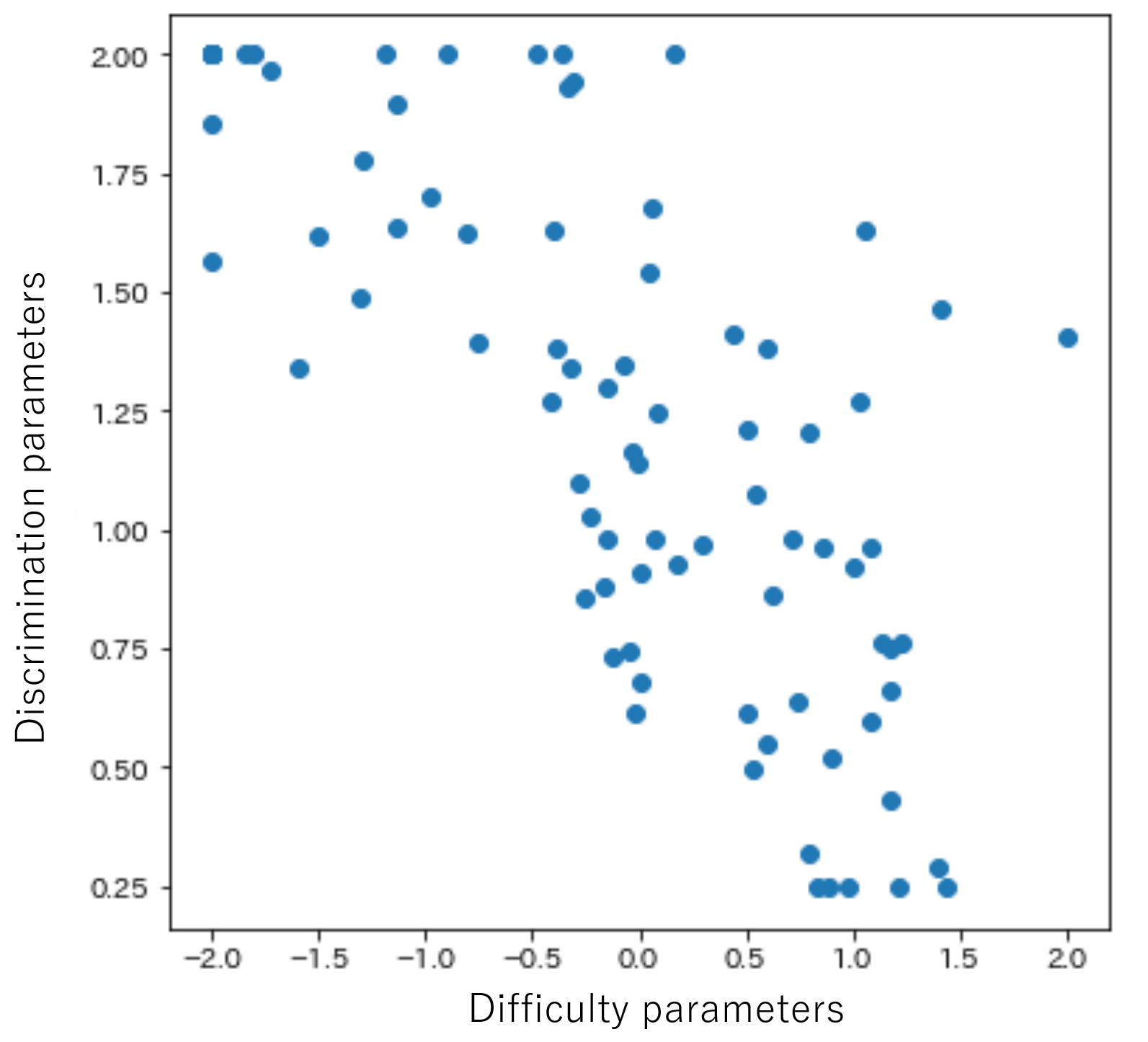Figure 7: The horizontal axis shows the discrimination parameters and the vertical axis shows the difficulty parameters.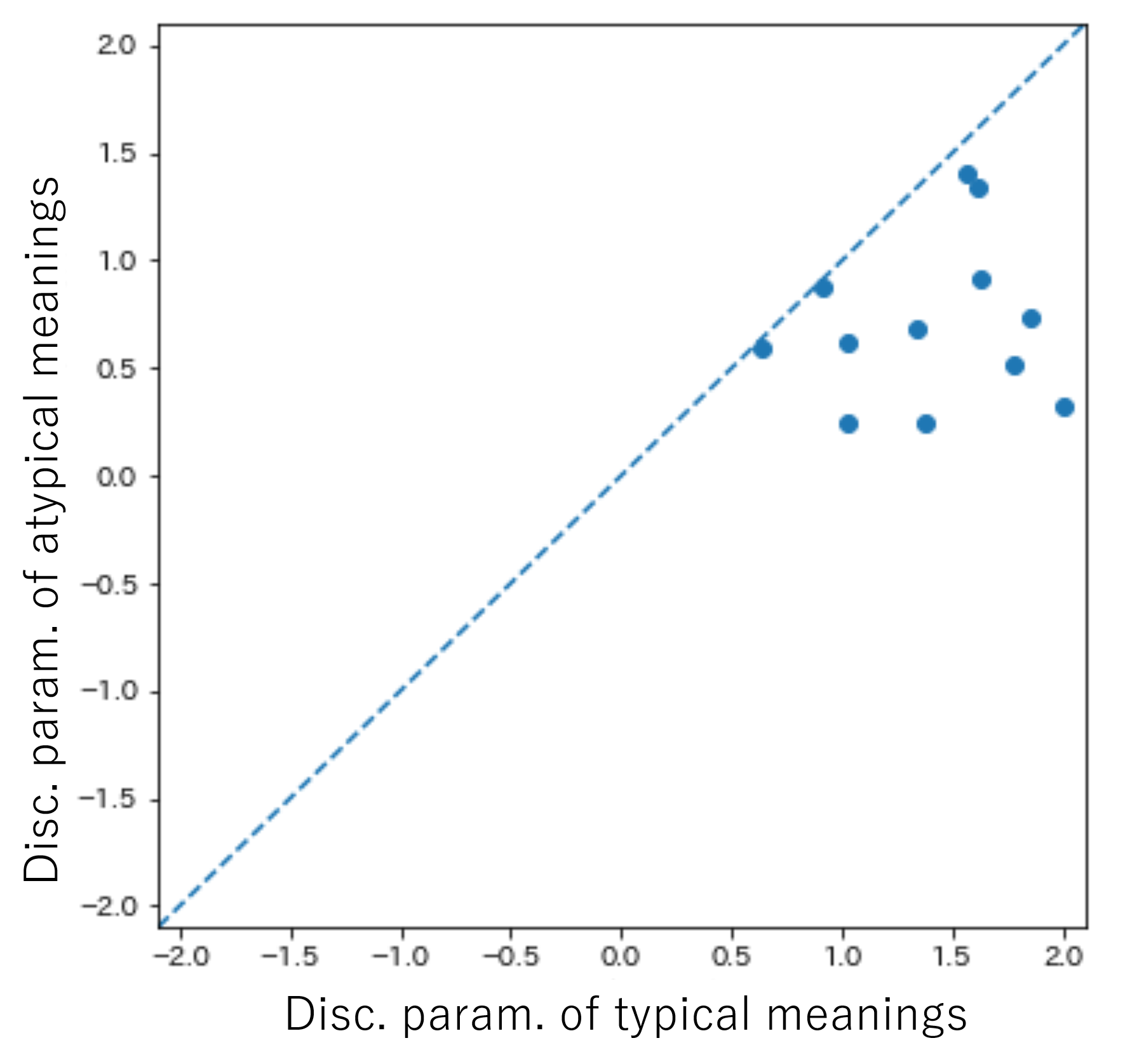Figure 8: The horizontal axis shows the discrimination parameter of the typical meanings and the vertical axis shows the discrimination parameter of atypical meaning.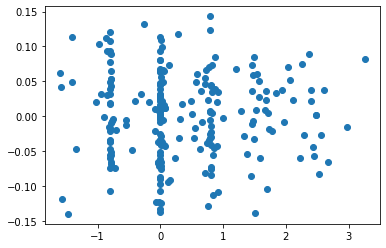Figure 9: Relationship between the learner ability parameter estimated by the pyirt software (horizontal) and the second principal component of the learner token embeddings (vertical)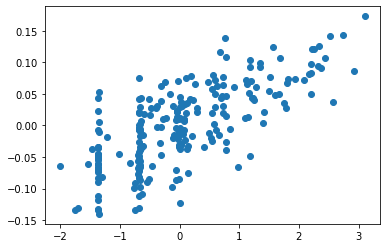Figure 10: Relationship between IRT ability parameters estimated by ltm on the R language (horizontal) and the first principal component of the learner token embeddings (vertical)